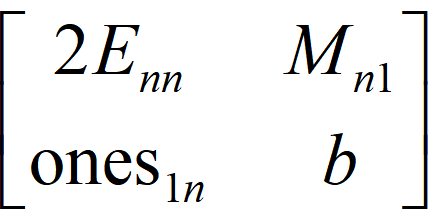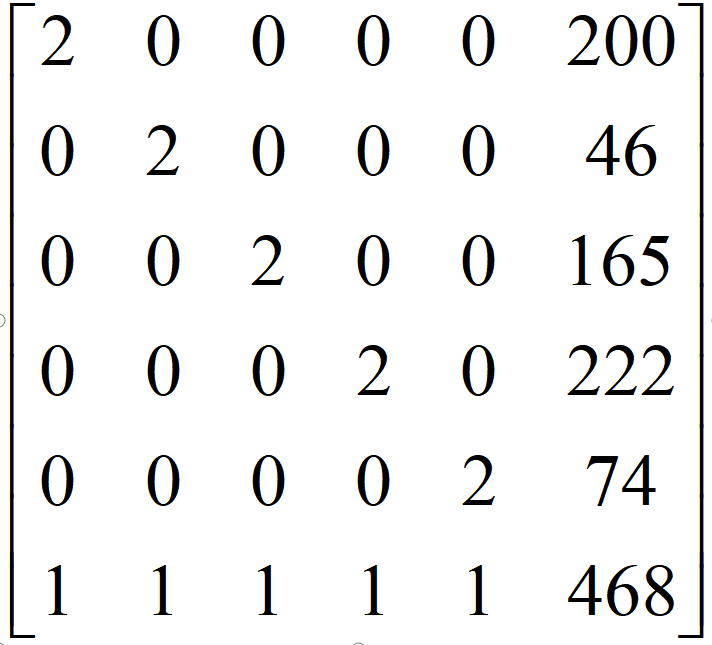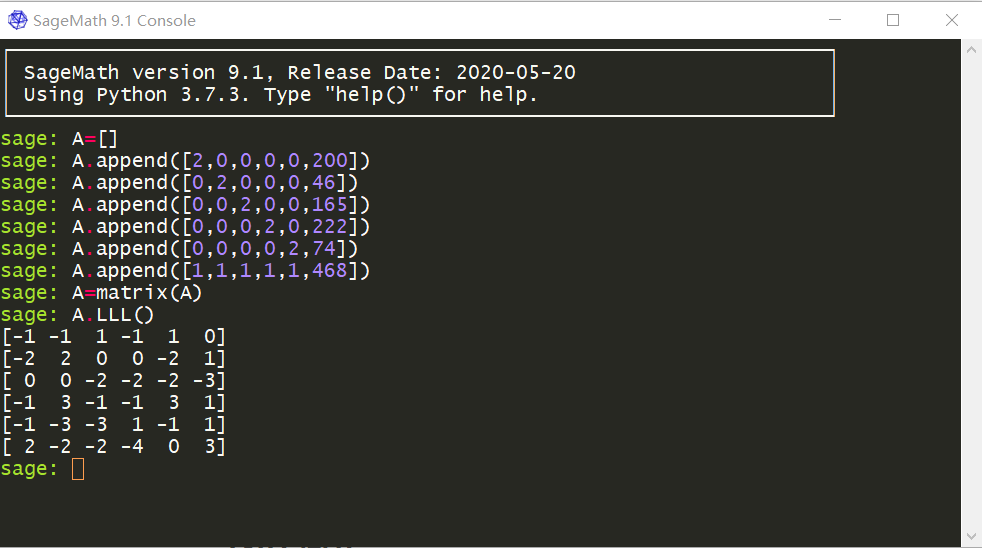# huangx607087学习格密码的笔记1

## 1.引入内容（Known -> Unknown）

### 1x01 简介

​ 众所周知，公钥密码学的安全性，往往建立在数学问题的困难性上。如 RSA 基于大数分解的困难性，DH 和 Elgamal 以及 ECC，是基于有限域上求离散对数的困难性。我们接下来要考虑一类新的难题，它们支撑了格（lattice）密码学。

对比起之前的公钥密码学体系，格密码提供了许多优势：首先，加密、解密速度可以更快（RSA 慢得难以忍受）；可以抵抗量子攻击。目前来看，暂且没有量子算法可以高效解决 lattice 难题。

​ 线性代数中讲过，在实数集$R$上有向量空间$V$,其中向量空间$V$是一部分向量的集合，并且任意两个向量相加，或者是某个向量的数乘，在$V$中仍然有意义。比如，假定$\vec{a},\vec{b} \in V$，则$\vec{a}+\vec{b},2\vec{a}$仍然在向量空间$V$内。

​ 而格实际上就与向量空间相似，不过对于格中的向量的数乘，我们仅限于乘上整数。

### 1x02 引例——一个新的密码系统

​ 我们先来看一个很简单的问题，而这个问题也开始与一个维度为$2$的格有所联系了。不过由于维度很低，它的安全性很弱，但它可以作为一个很恰当的引例，因为它可以说明如何在密码分析中出现晶格，即使潜在的硬问题似乎与晶格无关。 此外，它还提供了NTRU公钥密码系统的最低维介绍。

​ 加密开始，Alice先选择一个大的正整数$q$（不一定是素数）为一个公共参数作为公钥，并选择两个秘密的正整数$f,g$作为私钥，其中$f<\sqrt{\dfrac{q}{2}},\sqrt{\dfrac{q}{4}}<g<\sqrt{\dfrac{q}{2}}$且$\gcd(f,q)=1$。

​ 然后，Alice可以计算$h\equiv f^{-1}g\pmod q$。我们可以注意到$f,g$都小于$\sqrt q$，也就是说$f,g$的数量级是$O(\sqrt q)$的级别。而$h$的范围则是$O(q)$，在$q$很大的时候（比如$q>2^{256}$）时，$h$可以说是远大于$f,g$的。这个$h$也将作为公钥。随后，Alice将公钥$q,h$公开。

​ 作为信息发送方，Bob对信息加密的时候，也需要用到$2$个参数：明文$m$和一个随机数$r$，其中$m<\sqrt{\dfrac{q}{4}},r<\sqrt{\dfrac{q}{2}}$。然后他就可以计算密文$c\equiv rh+m \pmod q$。

​ 而收到密文后，Alice的解密过程如下：首先，他计算$a\equiv fc \pmod q$和$b\equiv ad \pmod g$，其中$d$是$f$在模$g$意义下的逆元。而神奇的是，对于$a= fc=frh+fm=frf^{-1}g+fm=rg+fm$。然后，我们可以得到$b=ad=drg+dfm\equiv dfm \pmod g$。又因为$df\equiv 1 \pmod g$，所以我们最后算出来的$b=m$就成立了。

## 2.子集问题和背包密码系统

### 2x03 一个新的公钥密码体系Merkle -Hellman Algorithm

MH-A算法是基于超级递增序列子集问题的一个公钥密码学体系，它使用同余进行伪装，然后通过创建公钥对和私钥对完成加密和解密的一系列操作。

Bob收到密钥之后，设明文为$\vec X$，其中$\vec X$是一个$n$维向量，每个分量取值为$0$或$1$。然后Bob计算$C=\vec X ·\vec M$完成加密。

Alice收到密文后，只需要计算出$D=A^{-1}C \pmod B$，然后求$D$在集合$\vec R$ 中的一个表示方法$\vec Q$即可解密。

Alice根据Bob的密文$C=468$，计算$D\equiv A^{-1}C\equiv 84×468 \equiv 41 \pmod {227}$。

### 2x04 对2x03中加密系统的攻击

2x03中我们提到的密码体系又称作子集公钥密码学系统或者背包密码学系统。其核心思想是生成一个超级递增序列，然后用模线性运算进行混淆，将混淆之后的序列作为公钥。### 2x05 LLL算法的初步实现 in Sagemath目录